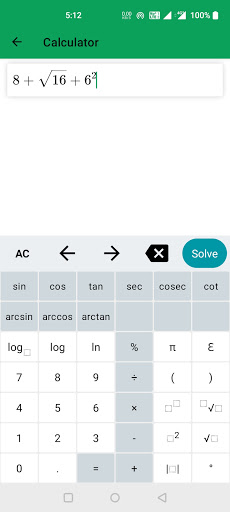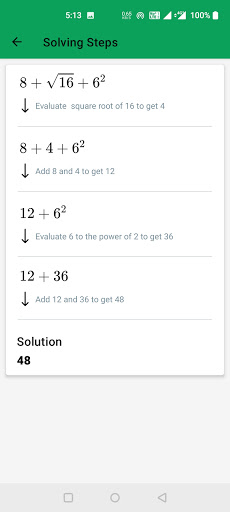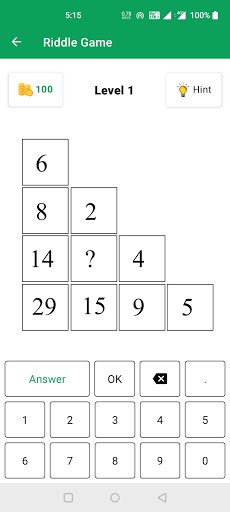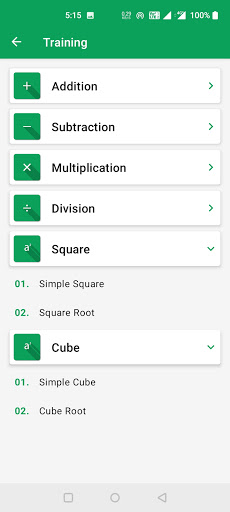Download inMath: Math Formula & Tricks on PC & Mac with AppKiwi APK Downloader

## AppKiwi - Download Mobile Apps on PC & Mac# inMath: Math Formula & Tricks on PC/Mac

Author: Hayatulerum

Score: 4.5

OS: Windows, OS X, 5.0 +##### About inMath: Math Formula & Tricks
inMath: Math Solver, is the best android application to learn mathematics. Math helps us think analytically and have better reasoning abilities. If you have not internet connection but even you can use this app without internet because its offline.

- Solve math problem and display step by step answer with explanations.

- It contains almost all mathematics formula for class 6th - 12th. This application is very useful to math solvers. Now math solvers will never forget any mathematical formulas.

- This app is also very useful for all students who wants to learn mathematics formula. Our app is made for every math lover. It has 1300+ mathematics formula in one app.

- It contains MCQ quiz for test yourself how much have you knowledge about math. It has quiz game for learning the math.

- It contains training option to speed up your calculation speed (e.g. addition, subtraction, multiplication etc.)

- It contains sample paper, previous question paper, NCERT books, NCERT solutions and Ncert Notes. You can simply download and read them. It will also help you in exam time.

- It has unit conversation option which is very helpful to you to convert units. Unit converter has all common which we use in our daily life.

- It has riddle game option to increase your thinking power. It helps to think analytically and have better reasoning abilities.

- It has a solve option which can solve some common functions like - Check BMI, Check Prime No, Find HCF, Solve Long Addition, Long Subtraction, Long Multiplication etc.

- This app does not collect any personal data.

If you liked our inMath, All Math Formula App, So please rate our application 5 🌟🌟🌟🌟🌟

Thankyou !!!!!

if you have any suggestion for us so contact us on this E-mail :- inmath@hayatulerum.com